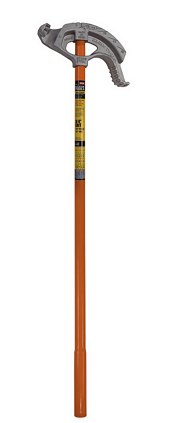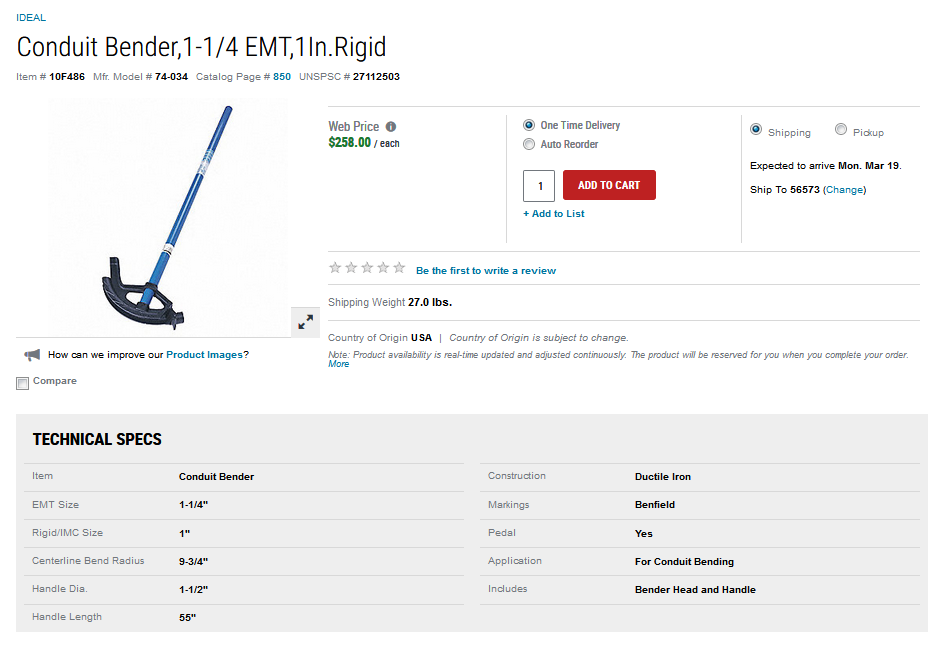# Electrical Conduit Math

Quote of the Day

A leader is a dealer in hope.

— Napoleon Bonaparte

As I have mentioned in other posts, I am building a large garage in northern Minnesota (Figure 1). I would show you some pictures of the interior, but I have promised my son that I will not post anything that could ruin his surprise when he sees it in April. As part of this construction effort, I am using quite a bit of electrical conduit. Conduit consists of metal pipes (often called EMT) through which the wires pass and it must be bent to go around any barriers it encounters. Conduit is a very efficient way to wire a working area because it directly attaches to the wall and does not require opening holes in drywall and repairing the damage. Conduit can also be updated and modified easily by running new/additional wires through it.

I am going to review the process for running conduit around an obstacle using a 4-point saddle bend, which entails bending the conduit into a trapezoidal shape for passing around the obstacle (Figure 1). Electrical handbooks contain tables that tell electricians how to measure along the conduit so that the bend will go around an object of a given depth. In this post, I will provide simple formulas for this bend and will use these formulas to regenerate a commonly seen table for conduit bending.

For those who like to follow along, my worksheet is here.

## Background

### Reference Article

In this post, I will duplicate a conduit bending table that I saw in this excellent reference article. The table is shown in Figure 3, which has units of degrees for angles and inches for length.

### Conduit Bending Video

Figure 4 shows a conduit bending video by a local trade school (Dunwoody) that I think is first-rate. The instructor covers both 4-point (trapezoid) and 3-point (triangular) bends. My focus in this post is the 4-point saddle bend because that is what I am dealing with in my garage construction right now.

 Figure 4: Good Briefing on 3-Point and 4-Point Saddle Bends.

### Conduit Bending Formulas

#### Conduit Bending Formulas Ignoring Bend Radius

There are two formulas that I need to generate: (1) shrinkage, which is the reduction in horizontal length caused by the bend; (2) bend distance, which is the horizontal length of the bend region. Figure 5 illustrates the geometry of the situation.

Applying basic trigonometry to Figure 5, we can derive Equations 1 and 2.

 Eq. 1$\displaystyle \text{BD }=\text{BO}\cdot \text{cot}\left( \theta \right)$ Eq. 2$\displaystyle \text{S}=\text{BO}\cdot \text{tan}\left( {\frac{\theta }{2}} \right)$

where

• BD, Bend Distance is the horizontal distance between bends.
• BO, Bend Offset is the depth of the obstacle to be passed over.
• Θ is the angle of the bend.
• S, Shrinkage is the effective reduction in horizontal conduit length because of the bend. Essentially, it is the difference in length between the hypotenuse and the base of a triangle.

I will use these equations to generate the table shown in Figure 3.

#### Conduit Bending Formulas Compensating for Bend Radius

Again, there are two formulas that I need to generate: shrinkage (Equation 3) and bend distance (Equation 5). An additional formula for the straight pipe length is also provided (Equation 4). Figure 6 illustrates the geometry of the situation and the associated formulas. The radius of the conduit bender, called R, will vary for each conduit bender. It normally is stamped on the bender, or the information is available in the vendor's literature.

Applying basic trigonometry to Figure 5, we can derive Equations 3, 4, and 5. Note that BD is defined slightly differently in that it represents the center-to-center distance between the bends.

 Eq. 3$\displaystyle S\text{ = }CL-HL=\left( {BO-4\cdot R} \right)\cdot tan\left( {\frac{\theta }{2}} \right)+2\cdot R\cdot \theta$ Eq. 4$\displaystyle SL=\left[ {BO-2\cdot R\cdot \left( {1-cos\left( \theta \right)} \right)} \right]\cdot \csc \left( \theta \right)$ Eq. 5$\displaystyle BD=R\cdot \theta +\left[ {BO-2\cdot R\cdot \left( {1-cos\left( \theta \right)} \right)} \right]\cdot \csc \left( \theta \right)$

Equations 3 - 5 are functions of the bend radius of the conduit bender. Because conduit benders can have different bend radii (see Figure 7), this means that using a single table for all conduit benders may result in some error – particularly for large bend offsets. Ideally, we would build a table for the conduit bender being used. I include this table with bend radius as a parameter on a worksheet in the Excel workbook associated with this post.Figure 7(a): Klein™ Conduit Bender with a 4" Bend Radius. Figure 7(b): Ideal™ Conduit Bender with a 5.25" Bend Radius.

## Analysis

### Approach

My focus here is on generating the traditional conduit bend table. In my workbook, I also include a tool using a more exact model.

There are a number of ways I could generate this table using Excel. The approach I chose was to:

• Generate a table of values for bend offsets of 1 inch. I call this my "reference table" because it is used for all subsequent calculations.
• Generate separate tables of shrinkage and bend distances.
• Collate shrinkage and bend distance columns by bend angle (Θ).

I chose this approach because I wanted to experiment with arranging columns by using a helper row containing the ordinal number of each column and doing a horizontal sort.

For demonstration purposes, I also included a tab where I used formulas to fill down the columns. A third tab was includes the conduit bender radius as a parameter.

### Reference Table

Figure 8 shows the shrinkage and bend distance formulas evaluated for a 1-inch bend offset (i.e. obstacle height), rounded to the nearest 1/16th of an inch. These values can be used as scale factors for other obstacle heights, which is exactly how the table in Figure 3 was generated.

### Full Table Generation

The table shown in Figure 3 is generated by multiplying the bend offsets by the scale factors in Figure 9. I used Excel tables to perform this action.

## Conclusion

I was able to duplicate the original table. I will be using this table for some conduit bending this weekend.

This entry was posted in Construction. Bookmark the permalink.

### 2 Responses to Electrical Conduit Math

1.Joshua wiliams says:

Hello,
I'm curious as to how you would mathemaitcally calculate shrink values in the instance of needing odd angles for the offset. Your page is very informative but it seems to be lacking how one would determine the R value which, I believe, is meant to represent the bend radius?
Also, does the abbreviation HL meant to be overall horizontal length?
Any information or further equations you could supplement would be a great help.
Thank you!

•mathscinotes says:

Thanks for taking the time to write. I appreciate when people help me clean things up. First, my page additions:

• I changed Figure 6 to define HL, which represents the horizontal distance.
• In the text, I added a definition of R as the conduit bender radius. This value is normally stamped on the conduit bender, mentioned on the packaging, or stated in the vendor documentation on their web site.

I frequently shop at Grainger and they often list the center-to-center bend radius in their product description (example below).As far as non-standard angles. Just go to the BendRadiusVersion tab and change the angle value in the table header to anything you want.

Again, thanks for helping me out.

mark